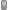CodeGuru Home VC++ / MFC / C++ .NET / C# Visual Basic VB Forums Developer.com

# Thread: Problem with get factored ?

1.MemberJoin Date
Oct 2006
Posts
123

## Problem with get factored ?

Hey ,

-------------

An integer that is greater than zero is said to be n-factored if it has n distinct factors other than 1 and itself. For example 12 is 4-factored because its factors are 2, 3, 4, 6. A prime number is 0-factored.
Write a function named isNFactored that takes two arguments, an integer and some value of n, and returns 1 if the integer is n-factored. The function signature is

int isNFactored(int value, int n)
Code:
```if value   n 	return	  reason
14	   2	 1	  14 has two factors, 2 and 7
18	   5	 0	  18 does not have 5 factors, it only has four (2, 3, 6 and 9)
25	   2	 0	  25 = 5*5 but we are only interested in distinct factors so the 5 is only counted once.
36	   7	 1	  36 has seven factors (2, 3, 4, 6, 9, 12, 18). Note that 6 is counted only once.
7	   0	 1	  all prime numbers are 0-factored
-5	  anything	  0	the value must be greated than 0
and I do code know but I doesn't know what is meant by return type?

public static int GetFactorCount(int numberToCheck, int n)
{
int factorCount = 0;
int sqrt = n;

// Start from 1 as we want our method to also work when numberToCheck is 0 or 1.
for (int i = 1; i < sqrt; i++)
{
if (numberToCheck % i == 0)
{
factorCount += 2; //  We found a pair of factors.
}
}

// Check if our number is an exact square.
if (sqrt * sqrt == numberToCheck)
{
factorCount++;
}

return factorCount;
}```Reply With Quote

2. ## Re: Problem with get factored ?

So: your method appears to count the number of factors (actually, there is a bug in this I think, but I will let you think about that) and then returns the number of factors that the numberToCheck has. Is this the information the method is supposed to return? (Hint: read the instructions)

Once you think you've gotten a correct solution, try passing it the values of each of your examples you listed above the method. If it returns the correct value, in the context of you already thinking it is error free, then you might consider yourself satisfied with the method. If it does not return the expected value, ask 'why' and step through the method to see what is going wrong. Common errors include:

-Syntax/semantic errors: You typed something other than what you actually meant
-Logic errors: You have made a mistake how the algorithm is supposed to operate. Think about what the method is supposed to do and check to see if it is doing that.

You're on the right track: keep going!

If you want to play with it some and then post with more targeted questions, please feel free.

I hope that helps!Reply With Quote

####Posting Permissions

• You may not post new threads
• You may not post replies
• You may not post attachments
• You may not edit your posts
•